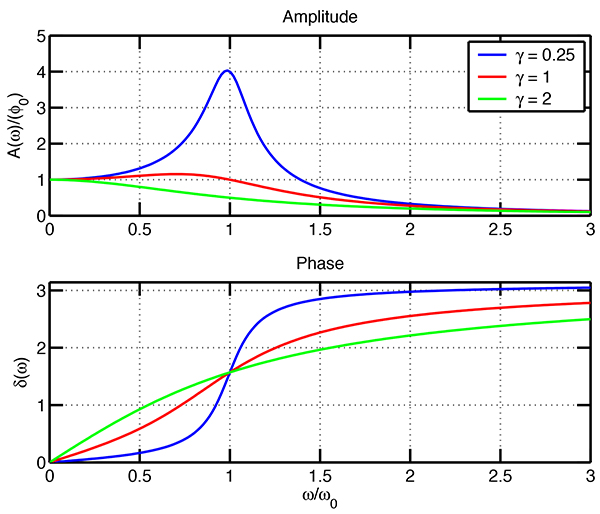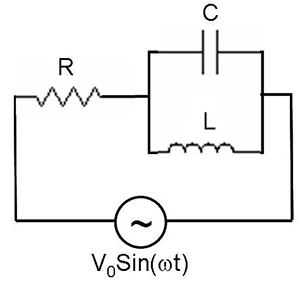RES.8-005 | Fall 2012 | Undergraduate
Vibrations and Waves Problem Solving
Driven Harmonic Oscillators

## Problems

### Driven Harmonic Oscillators

#### Problem 1

A torsional oscillator comprises a cylinder with moment of inertia, $$I$$, hanging from a light rod with torsional spring constant, $$\kappa$$. The cylinder also experiences a drag torque equal to $$-\mu \dot \theta$$, when moving with angular velocity $$\dot \theta$$. The top of the rod is driven with angular displacement $$\phi(t) = \phi_0 \cos{\omega t}$$.

1. Find the steady-state solution for $$\theta(t)$$.
2. Plot the amplitude $$A(\omega)$$ and phase $$\delta(\omega)$$ of your solution for $$\theta(t)$$ in (1) as a function of $$\omega$$. For your plot, assume that the natural frequency of oscillation of the system $$\omega_0 = 1$$, and plot three curves on the same plot with $$\frac{\mu}{I} = 0.25$$, 1 and 2. Label your curves to distinguish the three cases.

\begin{align*} \theta(t) &= \frac{\omega_0^2\,\phi_0}{\sqrt{\left(\omega_0^2 - \omega^2\right)^2 + \gamma^2\,\omega^2}}\,\cos\left({\omega\,t - \arctan{\left(\frac{\gamma\,\omega}{\left(\omega_0^2 -\omega^2\right)}\right)}}\right) \end{align*} \begin{align*} \text{where} \hspace{5mm} \gamma =\mu/I \hspace{5mm} \text{and} \hspace{5mm} \omega_0^2 = \kappa/I \end{align*}

\begin{align*} & A(\omega) = \frac{\omega_0^2\,\phi_0}{\sqrt{\left(\omega_0^2 - \omega^2\right)^2 + \gamma^2\,\omega^2}}\hspace{1mm}, \hspace{4mm} & \tan{\delta(\omega)} = \frac{\gamma\,\omega}{\left(\omega_0^2 -\omega^2\right)} \end{align*}#### Problem 2

A capacitor (of capacitance C), a resistor (of resistance R) and an inductor (of inductance L) are connected to an AC voltage source $$V = V_0 \sin(\omega t)$$ starting at $$t=0$$ as shown in the diagram below.Assuming that both the current and the charge of the capacitor are initially zero, determine the expression for $$V_C(t\ge0)$$ with $$\omega=\omega_0=\dfrac{1}{\sqrt{LC}}$$ and $$L < 4R^2C$$.